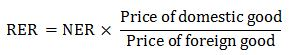Courses

# Exchange rates - Open Economy, Macroeconomics B Com Notes | EduRev

## B Com : Exchange rates - Open Economy, Macroeconomics B Com Notes | EduRev

The document Exchange rates - Open Economy, Macroeconomics B Com Notes | EduRev is a part of the B Com Course Macro Economics.
All you need of B Com at this link: B Com

#### Introduction to Nominal and Real Exchange Rates:

Exchange rates are of different types.

The usual distinction is between nominal exchange rate and real exchange rate.

The Nominal Exchange Rate:

The nominal exchange rate (NER) is the relative price of currencies of two countries. For example, if the exchange rate is £ 1 = \$ 2, then a British can exchange one pound for two dollars in the world market. Similarly, an American can exchange two dollars to get one pound.

The Real Exchange Rate:

The real exchange rate (RER) refers to the relative price of goods of Britain and USA. It is the rate at which the Britishers can trade its own goods for those of the USA. The real rate is another name for the terms of trade, which is expressed as Px/Pm, where Px is the price of export and Pm is the price of import.

The real exchange rate is expressed as:This means that the rate at which the British can exchange foreign and domestic goods depends on two factors:

(i) The price of the good in local currency; and

(ii) The rate at which the two currencies are exchanged.

Symbolically, the RER may be expressed as: er = ex (P/ P*)

where er is the RER, en is the NER and (P/ P*) is the ratio of price level in the home country (P) and the price level in the foreign country (P*).

What is the Implication of RER?

A high (low) RER implies that foreign goods are relatively cheap (expensive) and domestic goods are relatively expensive (cheap).

The Real Exchange Rate and Balance of Trade:

The RER is just a relative price. This means that the relative demand for domestic and foreign goods is affected by the relative price of two sets of goods.

If, initially, RER is low, domestic residents (say, UK buyers) will buy few imported (USA) goods. For exactly the same reason, foreigners (say, US buyers) will want to buy more of domestic (UK goods). For both the reasons, the demand for Britain’s net exports will be high.

The converse is also true. If RER is high, domestic residents (the Britishers) will want to buy many imported goods and foreigners (the Americans) will want to buy few British goods. So the quantity of America’s net exports demanded will be very much on the low side.

Thus we can write the functional relationship between RER and NX as:

NX = NX(er)

The inverse relationship between the BOT and the RER is shown in Fig. 6.5. We see that at initial RER (er0), trade is balanced, i.e., X = M. At a higher RER, er2, there is a deficit in BOT. And at a lower RER, er1, there is a surplus in BOT.

#### The Determinants of the Nominal Rate of Exchange:

So long we were concerned with the determination of the RER and its effect on trade balance and social welfare. Now we examine how the nominal exchange rate (NER) is determined. When we refer to the “exchange rate” between two countries, we usually mean the NER.

The NER is written as:

e = ex (P*/P)

It is the rate at which the currencies of two countries are exchanged.

The above equation shows that NER depends on the RER and the price levels in the two countries. Given the value of RER, if the domestic price level P rises, then the RER will fall. Since a pound is worth less, it will buy fewer dollars. However, if the US price level P* rises, then the NER will go up. This means that the dollar is worth less, a pound will buy more dollars.

Since the foreign exchange market is dynamic, economists find it interesting to study exchange rate movements over time.

The above equation can now be expressed as:

Percentage change in e = % change in er + % change in P* – % change in P

= % change in er + % change in π* – % change in π

= % change in er + (π* – π), where π is the domestic inflation and π* is the foreign country’s inflation rate.

Thus, percentage change in NER between pound and dollar equals the percentage change in RER plus the difference in rates of inflation in the two countries.

If a foreign country (the USA) has a higher (lower) rate of inflation than UK (the home country) a pound will buy a larger (smaller) amount of a foreign currency (dollar) over time.

Thus, we find that NER is a purely monetary phenomenon. It is affected by monetary policy, which determines the rate of domestic inflation. The Quantity Theory of Money, studied in chapter 4, suggests that a certain percentage increase in money supply will lead to proportionate increase in the aggregate price level.

There is an inverse relation between domestic inflation rate and the NER. High inflation (π) in Britain leads to depreciation of the pound, i.e., a fall in e. Alternatively stated, just as an increase in money supply raises the prices of goods measured in terms of domestic currency, it also raises the price of a foreign currency (dollar) measured in terms of the domestic currency (pound).

Offer running on EduRev: Apply code STAYHOME200 to get INR 200 off on our premium plan EduRev Infinity!

## Macro Economics

61 videos|61 docs

,

,

,

,

,

,

,

,

,

,

,

,

,

,

,

,

,

,

,

,

,

,

,

,

;